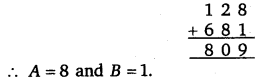# Class 8 Maths NCERT Solutions for Chapter – 16 Playing with Numbers Ex – 16.1

## Playing with Numbers

Question 1.
Find the values of the letters in each of the following and give reasons for the steps involved.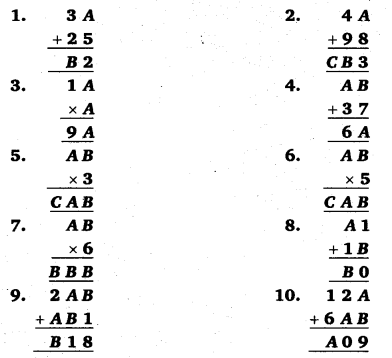Solution:
1. There are two letters whose values are to be found out.
Study the addition in the ones column, i. e., from A + 5 we get 2, i. e., the number whose ones digit is 2.
For this to happen, A must be 7 (∵ A + 5 = 7 + 5 = 12).
So for the addition in tens column, we have
1 + 3 + 2 = B ⇒ B = 6
So, the puzzle has been decoded as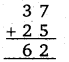∴ The possible values of A and B are 7 and 6 respectively.

2. There are three letters whose values are to be found out.
Study the addition in the ones column, i. e., from A + 8 we get 3, i. e., the number whose ones digit is 3.
For this to happen, A must be 5 (∵ A + 8 = 5 + 8 = 13).
So for the addition in tens column, we have 1 + 4 + 9 = B ⇒ 14 = B
∴Clearly, B is 4 and C is 1.
So the puzzle has been decoded as∴ The possible values of A, B and C are 5, 4 and 1 respectively.

3. Since the ones digit of A x A is A, it must be that A = 1 or A = 5 or A = 6.4. There are two letters whose values are to be found : A and B.
Study the addition in the given puzzle. .
i. e., from B + 7 we get A and from A + 3 we get 6
Possible values can be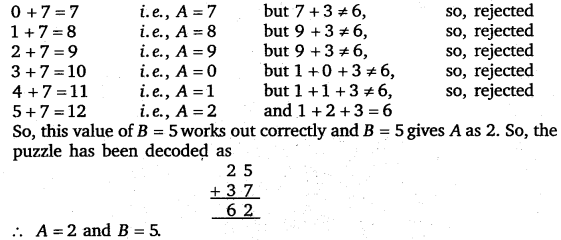5. This has three letters whose values are to be found : A, B and C.
Since the ones digit of 3 x B = B, it must be B = 0. So, the puzzle becomes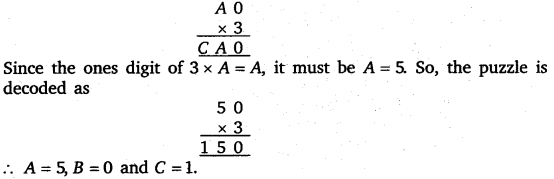6. This has three letters whose values are to be found: A, B and C. Since the ones digit of 5 x B is B, it must be that B = 0 or B = 5 If B = 0, then the puzzle becomes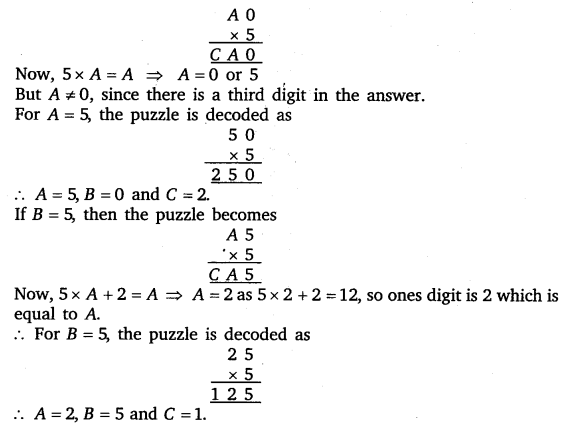7. This has two letters whose values are to be found : A and B.Possible values of BBB are 111, 222, 333, etc.
Let us divide these numbers by 6.
111 ÷ 6 =18, remainder 3. So, 111 is rejected.
222 ÷ 6 = 37, remainder 0. So, the quotient 37 is not of the form A2. Thus,
222 is rejected.
333 ÷ 6 = 55, remainder 3. So, 333 is rejected.
444 ÷ 6 = 74, remainder 0. The quotient 74 is of the form A4.
So, the puzzle has been decoded as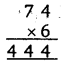This one clearly works out correctly.
So, the answer is A = 7 and B = 4.

8. This has two letters whose values are to be found : A and B.
Study the addition in the ones column : from 1 + B we get 0, that is, a number whose ones digit is 0.
For this to happen, the ones digit of B should be 9. And since B itself is a digit, we get B =9. So, the puzzle becomes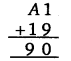But 90 – 19 = 71 so, A1 = 71 ⇒ A = 7
Thus, A = 7 and B = 9 is the answer.

9. There are two letters whose values are to be found out.
Study the addition in the ones column, i. e., from B + 1 we get 8, that is, a number whose ones digit is 8.
For this to happen, the ones digit of B should be 7. As B itself is a digit, we get B = 7. So the puzzle becomes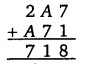Now study the addition in the tens column, i. e., from A+ 7 we get 1, that is, a number whose ones digit is 1.
For this to happen, the ones digit of A should be 4. As A itself is a digit, we get A = 4. So, the puzzle has been decoded asTherefore, possible values of A and B are 4 and 7 respectively.

10. This has two letters whose values are to be found : A and B.
Study the addition in the tens column : from 2 + A we get 0, that is, a number whose ones digit is 0.
For this to happen, the ones digit of A should be 8. And since A is a digit, we get A = 8. So, the puzzle becomes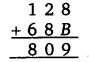Now, study the addition in the ones column: from 8 + B we get 9. For this to happen, we must have B = 1. Therefore, the puzzle has been decoded as